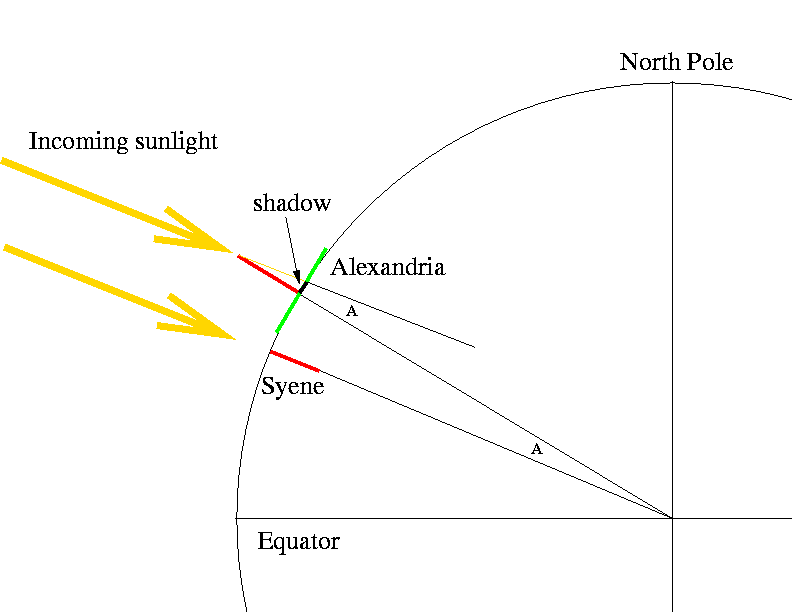# Astronomy 101 Specials: Eratosthenes and the Size of the Earth

Eratosthenes' method for determining the size of the Earth was an elegant application of simple geometry to an otherwise very difficult problem. By using the difference in the elevation of the noontime sun at two different locations, he was able to measure the angular difference betwen the vertical directions at those two locations. This angular difference told him what fraction of the way around the earth separated the two locations. He then used this fraction and the measured distance between the two locations to estimate the distance around the earth (a.k.a. the circumference).

As the story goes, old Eratosthenes learned that at noon on one day a year (the summer solstice, but that's not really important), the Sun shone directly down a deep vertical well in a town called Syene, about 500 miles south of his home in Alexandria. Eratosthenes reasoned that the Sun must be directly overhead at noon in Syene at this time. He knew that the Sun was not directly overhead in Alexandria on this day, so he figured that the vertical direction in Alexandria was different from the vertical direction in Syene.

This conclusion was based on the assumption that the Sun was far enough away (compared to the distance between Syene and Alexandria) that the rays of incoming sunlight at both locations were parallel.So how different were the vertical directions at the two locations? Well, he decided to measure this with a vertical stick in the ground called a gnomon. He watched the shadow of the gnomon on the day in question, and measured the length of the shadow at noon. The height of the stick and the length of the shadow allowed him to calculate the angle between the vertical at Alexandria (as indicated by the gnomon) and the direction of the Sun's rays at noon.This angle, labeled ``A'' in the above drawing, is calculated as follows:

tan(A) = (length of shadow)/(height of gnomon)

where tan(A) is the tangent function, which was well-known at the time. By calculating this angle, Eratosthenes had calculated the angular difference between the verticals at Syene and Alexandria. Why? Because the sunward direction is the vertical direction at Syene on this day. You can see this graphically in the figure above, by noting that the sunward directions (yellow lines) are parallel, and therefore that the two angles labeled ``A'' really have the same value. (Remember? A line crossing two parallel lines makes the same angle with each line?)

So Eratosthenes made this measurement and found that the value for angle ``A'' is 7.2 degrees. He also knew that the actual distance between Alexandria and Syene was 5040 stades (1 stade = about 160 m) because somebody had measured it out by foot. Well 7.2 degrees is only 7.2/360ths of the way around the globe (since all the way around is 360 degrees). If that distance is 5040 stades, then the total distance around the earth must be

• total distance around the earth = 5040 stades x (360 degrees)/(7.2 degrees)
• = 5040 x 50 stades
• = 250,000 stades or 2.5 x 105 stades.

Back to the Astronomy 101 Specials Page